## Bitwise OperatorsS2C Home « Bitwise Operators

In the second lesson on operators we look at the bitwise logical and bitwise shift operators. Bitwise operators perform their operations on the integer types `byte`, `short`, `int` and `long` and will not work with any other type. These operators are used to manipulate the bits within an integer value, hence the name.

• Bits with the value `0` are said to be switched off.
• Bits with the value `1` are said to be switched on.
• All bitwise conversions get promoted to the `int` type before conversion so you need to cast back to `short` and `byte` when using these types for the target.

### Bitwise Logical Operators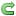Top

The bitwise logical operators perform the same operations as the logical operators discussed in the last lesson but work on a bit-by-bit basis on the integer type. The following table shows all possible combinations for a bit using `0` and `1`.

Operator Meaning Example Result Notes
&`AND`bit a = 0 and bit b = 0
a & b
bit a = 0 and bit b = 1
a & b
bit a = 1 and bit b = 0
a & b
bit a = 1 and bit b = 1
a & b

`0`

`0`

`0`

`1`
Will turn the result bit to `0` unless both bits are `1`.

Useful for switching bits off.
|`OR`bit a = 0 and bit b = 0
a | b
bit a = 0 and bit b = 1
a | b
bit a = 1 and bit b = 0
a | b
bit a = 1 and bit b = 1
a | b

`0`

`1`

`1`

`1`
Will turn the result bit to `1` if either bits are `1`.

Useful for switching bits on.
^`XOR` (exclusive `OR`)bit a = 0 and bit b = 0
a ^ b
bit a = 0 and bit b = 1
a ^ b
bit a = 1 and bit b = 0
a ^ b
bit a = 1 and bit b = 1
a ^ b

`0`

`1`

`1`

`0`
Will switch the result bit to `1` if only one of the bits is `1` otherwise the result bit is switched to 0.

Useful for highlighting unmatched bits.
~`NOT`bit a = 0
~a
bit a = 1
~a

`1`

`0`
Useful for switching bits to show the compliment of the number.

Let's look at each of the bitwise logical operators in turn to get a feel for how they work.

#### Bitwise ANDTop

The bitwise AND operator can be used for turning non-matched bits off. Lets look at some code to illustrate how this works:

``````
/*
Bitwise AND
*/
public class BitwiseAnd {

public static void main (String[] args) {
byte a = 97;
printBits(a);
byte b = 57;
printBits(b);
byte c = (byte) (a & b);
printBits(c);
}

/*
Method to loop through input parameter and print out the bits
*/
static void printBits (byte aByte) {
System.out.print("Input param aByte = " + aByte + ": ");
for (int i = 128; i > 0; i /= 2) {
if ((aByte & i) != 0) {
System.out.print("1 ");
} else {
System.out.print("0 ");
}
}
System.out.println(" ");
}
}

``````

Save, compile and run the file in directory   c:\_BeginningJava5Ok there are several new things going on with the above piece of code that we will go through. The first thing to notice is we are casting to a byte when using the bitwise AND (`byte c = (byte) (a & b);`). All bitwise conversions get promoted to the `int` type before conversion so we need to cast back to the `byte` type. Also we are enclosing the bitwise operation in parentheses so the whole expression gets evaluated and not just the `a`. We are using the `printBits` method to save replication of code. We pass each variable to this method and print the value. We then use a `for` loop to print out each bit of the byte variable passed to the method. We will go through the `for` loop in the Loop Statements lesson but what we are doing here is just dividing 256 by 2 to get to each bit. The 8th bit is used for the sign (0 = positive, 1 negative), hence the actual value range of a byte is `127` to `-128` as stated in the Primitive Variables lesson. As you can see from the output the bitwise AND operator switches non-matched bits off.

#### Bitwise ORTop

The bitwise OR operator can be used for turning non-matched bits on. Lets look at some code to illustrate how this works:

``````
/*
Bitwise OR
*/
public class BitwiseOr {

public static void main (String[] args) {
byte a = 97;
printBits(a);
byte b = 57;
printBits(b);
byte c = (byte) (a | b);
printBits(c);
}

/*
Method to loop through input parameter and print out the bits
*/
static void printBits (byte aByte) {
System.out.print("Input param aByte = " + aByte + ": ");
for (int i = 128; i > 0; i /= 2) {
if ((aByte & i) != 0) {
System.out.print("1 ");
} else {
System.out.print("0 ");
}
}
System.out.println(" ");
}
}

``````

Save, compile and run the file in directory   c:\_BeginningJava5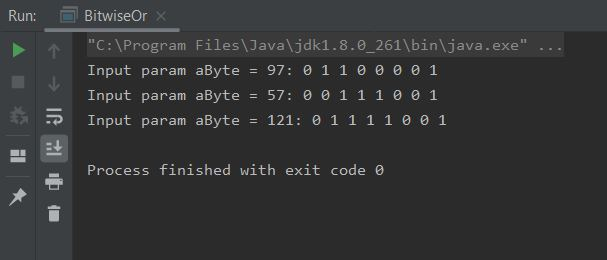As you can see from the output the bitwise OR operator switches non-matched bits on.

#### Bitwise XORTop

The bitwise XOR operator can be used for highlighting unmatched bits as any matching bits will be switched to `0`, whilst unmatched bits are switched to `1`. Lets look at some code to illustrate how this works:

``````
/*
Bitwise XOR
*/
public class BitwiseXor {

public static void main (String[] args) {
byte a = 97;
printBits(a);
byte b = 57;
printBits(b);
byte c = (byte) (a ^ b);
printBits(c);
}

/*
Method to loop through input parameter and print out the bits
*/
static void printBits (byte aByte) {
System.out.print("Input param aByte = " + aByte + ": ");
for (int i = 128; i > 0; i /= 2) {
if ((aByte & i) != 0) {
System.out.print("1 ");
} else {
System.out.print("0 ");
}
}
System.out.println(" ");
}
}

``````

Save, compile and run the file in directory   c:\_BeginningJava5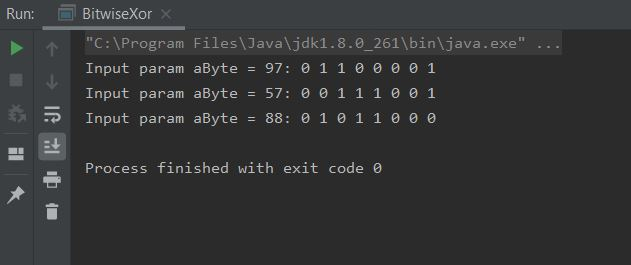As you can see from the output the bitwise XOR operator switches non-matched bits on and matched bits off.

#### Bitwise NOTTop

The bitwise NOT operator can be used for switching bits to show the compliment of the number. Lets look at some code to illustrate how this works:

``````
/*
Bitwise NOT
*/
public class BitwiseXor {

public static void main (String[] args) {
byte a = 57;
printBits(a);
a = ~a;
printBits(a);
}

/*
Method to loop through input parameter and print out the bits
*/
static void printBits (byte aByte) {
System.out.print("Input param aByte = " + aByte + ": ");
for (int i = 128; i > 0; i /= 2) {
if ((aByte & i) != 0) {
System.out.print("1 ");
} else {
System.out.print("0 ");
}
}
System.out.println(" ");
}
}

``````

Save, compile and run the file in directory   c:\_BeginningJava5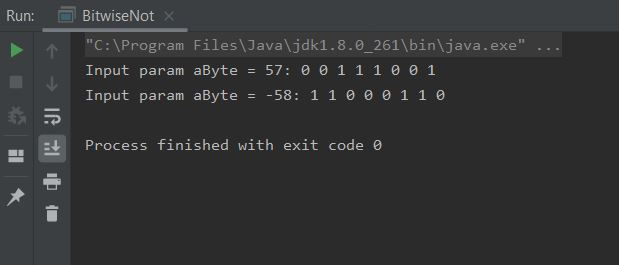As you can see from the output the bitwise NOT operator switches bits to show the compliment of the number.

### Bitwise Shift OperatorsTop

The bitwise shift operators allow us to shift the bits that make up an integer type to the left or right by a specified amount.

Operator Meaning Example Result Notes
<<`Left shift``int a = 1234; a = a << 2;``4936`Will shift the value by the specified number of bits to the left.

value << numberOfBits.
>>`Right shift``int a = 1234; a = a >> 3;``154`Will shift the value by the specified number of bits to the right.

value >> numberOfBits.
>>>`Signed right shift``int a = -12345; >>> 4;``-772`Will shift the value by the specified number of bits to the right, whilst retaining the signed bit.

If the value is negative then the resultant moved bits will be sign-extended.

value >>> numberOfBits.

Let's look at each of the bitwise shift operators in turn to see how we got the values in the table above.

#### Bitwise Left ShiftTop

The bitwise left shift operator allows us to shift the bits in an integer type to the left by the specified amount.

``````
/*
Bitwise Left Shift
*/

public class BitwiseLeftShift {

public static void main (String[] args) {
short a = 1234;
printBits(a);
a = (short) (a <<  2);
printBits(a);
}

/*
Use a loop to print out the bits
*/
static void printBits (short aShort) {
System.out.print("Input param aShort = " + aShort + ": ");
for (int i = 32768 ; i > 0; i /= 2) {
if (i == 128) {
System.out.print(" |  ");
}
if ((aShort & i) != 0) {
System.out.print("1 ");
} else {
System.out.print("0 ");
}
}
System.out.println(" ");
}
}

``````

Save, compile and run the file in directory   c:\_BeginningJava5As you can see from the output all the bits have been shifted 2 places to the left giving us a new value. We are using the `|` symbol to split the result into 8-bit chunks.

#### Bitwise Right ShiftTop

The bitwise right shift operator allows us to shift the bits in an integer type to the right by the specified amount.

``````
/*
Bitwise Right Shift
*/

public class BitwiseRightShift {

public static void main (String[] args) {
short a = 1234;
printBits(a);
a = (short) (a >>  3);
printBits(a);
}

/*
Use a loop to print out the bits
*/
static void printBits (short aShort) {
System.out.print("Input param aShort = " + aShort + ": ");
for (int i = 32768 ; i > 0; i /= 2) {
if (i == 128) {
System.out.print(" |  ");
}
if ((aShort & i) != 0) {
System.out.print("1 ");
} else {
System.out.print("0 ");
}
}
System.out.println(" ");
}
}

``````

Save, compile and run the file in directory   c:\_BeginningJava5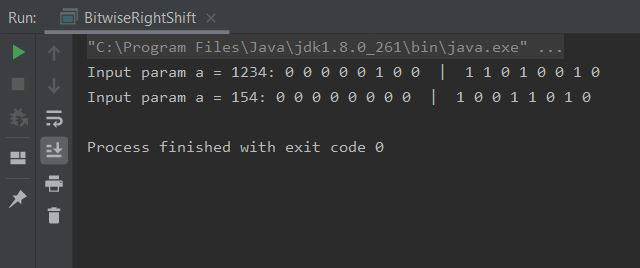As the output shows all the bits have been shifted 3 places to the right giving us a new value. We are using the `|` symbol to split the result into 8-bit chunks.

#### Bitwise Signed Right ShiftTop

The bitwise signed right shift operator allows us to shift the bits in an integer type to the right by the specified amount, whilst retaining the signed bit.

``````
/*
Bitwise Signed Right Shift
*/

public class BitwiseSignedRightShift {

public static void main (String[] args) {
short a = -12345;
printBits(a);
a = (short) (a >>>  4);
printBits(a);
}

/*
Use a loop to print out the bits
*/
static void printBits (short aShort) {
System.out.print("Input param aShort = " + aShort + ": ");
for (int i = 32768 ; i > 0; i /= 2) {
if (i == 128) {
System.out.print(" |  ");
}
if ((aShort & i) != 0) {
System.out.print("1 ");
} else {
System.out.print("0 ");
}
}
System.out.println(" ");
}
}

``````

Save, compile and run the file in directory   c:\_BeginningJava5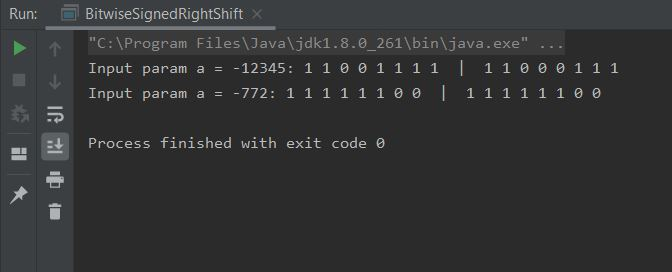As the output shows all the bits have been shifted 3 places to the right giving us a new value. We are using the `|` symbol to split the result into 8-bit chunks.

Did you spot anything else? When you right shift a negative number, when the interim expression is promoted to an `int` from a `short` or `byte` all the extra bits are sign-extended. Thus when we cast back to a `short` in our example all bits shifted in after our signed bit are `1` and we get an unexpected result. Be aware of this when using this particular bitwise operator.

### Bitwise Shorthand Assignment OperatorsTop

The bitwise shorthand assignment operators allow us to write compact code that is implemented more efficiently. When using the bitwise shorthand assignment operators there is no need to cast either.

Operator Meaning Example Result Notes
&=Bitwise AND`byte a = 74; a &= 124;`72Will give the Bitwise AND result of 74 and 124.
|=Bitwise OR`byte a = 74; a |= 124;`126Will give the Bitwise OR result of 74 and 124.
^=Bitwise XOR`byte a = 74; a ^= 124;`54Will give the Bitwise XOR result of 74 and 124.
<<=Left shift`short a = 1234; a <<= 2;`4936Left shift 1234 by 2 bits.
>>=Right shift`short a = 1234; a >>= 3;`154Right shift 1234 by 3 bits.
>>>=Signed right shift`short a = -12345; a >>>= 4;`-772Signed right shift -12345 by 4 bits.

### Operator PrecedenceTop

We will finish our discussion on operators with a table showing the order of precedence that is invoked when Java interprets operator symbols. The list includes the special operators which will be discussed in later lessons. When using multiple operators in an expression it is always best practice to use parentheses to explicitly state the order of precedence for clarity and readability. The table lists order of precedence from highest to lowest.

Precedence Operators Operation Associativity
1`()`method callleft
`[ ]`array index
`.`method access
2`+`unary plusright
`-`unary minus
`++`prefix/postfix increment
`--`prefix/postfix decrement
`!`boolean logical NOT
`~`bitwise NOT
`(type)`type cast
`new`object creation
3`/`divisionleft
`*`mutiplication
`%`modulus
4`+`addition or string concatenationleft
`-`subtraction
5`<<`bitwise left shiftleft
`>>`bitwise right shift
`>>>`bitwise signed right shift
6`/`less thanleft
`<=`less than or equal to
`>`greater than
`>=`greater than or equal to
`instanceof`reference test
7`==`equal toleft
`!-`not equal to
8`&`boolean logical AND or bitwise ANDleft
9`^`boolean logical XOR or bitwise XORleft
10`|`boolean logical OR or bitwise ORleft
11`&&`short-cricuit boolean logical ANDleft
12`||`short-cricuit boolean logical ORleft
13`? :`conditionalright
14`=`assignmentright
`+=`shorthand addition
`-=`shorthand subtraction
`/=`shorthand division
`*=`shorthand mutiplication
`%=`shorthand modulus
`&=`shorthand boolean logical AND or bitwise AND
`|=`shorthand boolean logical OR or bitwise OR
`^=`shorthand boolean logical XOR or bitwise XOR
`<<=`shorthand bitwise left shift
`>>=`shorthand bitwise right shift
`>>>=`shorthand bitwise signed right shift

## Lesson 6 Complete

In this lesson we took a second look at the symbols used in Java5 for mathematical and logical manipulation.

### What's Next?

In the next lesson we look at the conditional statements available for use in Java5.